# 模板匹配

### 方法

cv2.matchTemplate(img, template, methods)

• TM_SQDIFF：计算平方不同，计算出来的值越小，越相关
• TM_CCORR：计算相关性，计算出来的值越大，越相关
• TM_CCOEFF：计算相关系数，计算出来的值越大，越相关
• TM_SQDIFF_NORMED：计算归一化平方不同，计算出来的值越接近0，越相关
• TM_CCORR_NORMED：计算归一化相关性，计算出来的值越接近1，越相关
• TM_CCOEFF_NORMED：计算归一化相关系数，计算出来的值越接近1，越相关
具体公式可参考：Opencv官网

## 举例说明：img = cv2.imread('lena.jpg', 0)
h, w = template.shape[:2]

methods = ['cv2.TM_CCOEFF', 'cv2.TM_CCOEFF_NORMED', 'cv2.TM_CCORR',
'cv2.TM_CCORR_NORMED', 'cv2.TM_SQDIFF', 'cv2.TM_SQDIFF_NORMED']
# 先使用计算平方的方法匹配模板
res = cv2.matchTemplate(img, template, cv2.TM_SQDIFF)
# 得到计算值的最大值、最小值以及它们的左上角的位置
min_val, max_val, min_loc, max_loc = cv2.minMaxLoc(res)
# 运用所有方法
for meth in methods:
img2 = img.copy()

# 匹配方法的真值
method = eval(meth)
res = cv2.matchTemplate(img, template, method)
min_val, max_val, min_loc, max_loc = cv2.minMaxLoc(res)

# 如果是平方差匹配TM_SQDIFF或归一化平方差匹配TM_SQDIFF_NORMED，取最小值；否则取最大值。
if method in [cv2.TM_SQDIFF, cv2.TM_SQDIFF_NORMED]:
top_left = min_loc
else:
top_left = max_loc
bottom_right = (top_left + w, top_left + h)

# 画矩形
cv2.rectangle(img2, top_left, bottom_right, 255, 2)

plt.subplot(121), plt.imshow(res, cmap='gray')
plt.xticks([]), plt.yticks([])  # 隐藏坐标轴
plt.subplot(122), plt.imshow(img2, cmap='gray')
plt.xticks([]), plt.yticks([])
plt.suptitle(meth)
plt.show()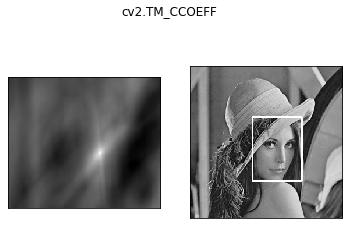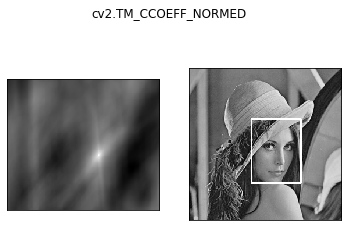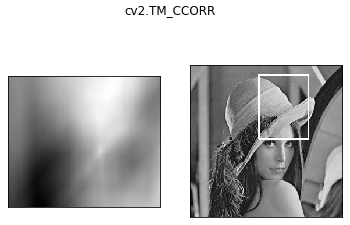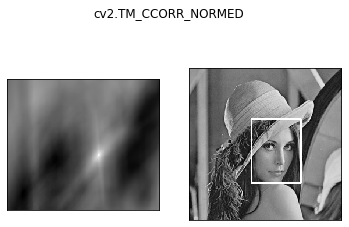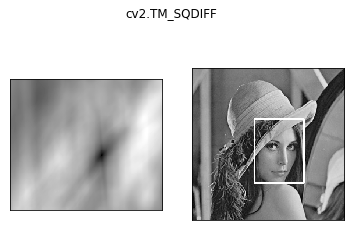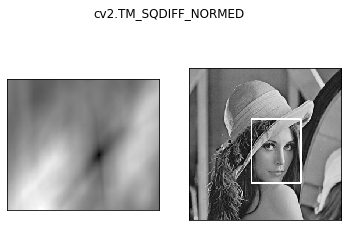## 匹配多个对象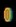img_rgb = cv2.imread('mario.jpg')
img_gray = cv2.cvtColor(img_rgb, cv2.COLOR_BGR2GRAY)
h, w = template.shape[:2]

res = cv2.matchTemplate(img_gray, template, cv2.TM_CCOEFF_NORMED)
threshold = 0.8
# 取匹配程度大于%80的坐标，而不是找匹配度最大的坐标。
loc = np.where(res >= threshold)
for pt in zip(*loc[::-1]):  # *号表示可选参数；[:,:,-1]表示调转loc中坐标的顺序，比如原来是(40, 69)，现在变成(69, 40)。
bottom_right = (pt + w, pt + h)
cv2.rectangle(img_rgb, pt, bottom_right, (0, 0, 255), 2)

cv2.imshow('img_rgb', img_rgb)
cv2.waitKey(0)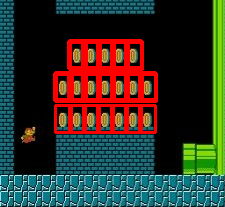## PS: np.where()函数的用法

#### 方法1：

np.where(condition, x, y)

#### 方法2：

np.where(condition)

©️2019 CSDN 皮肤主题: 护眼 设计师: 闪电赇